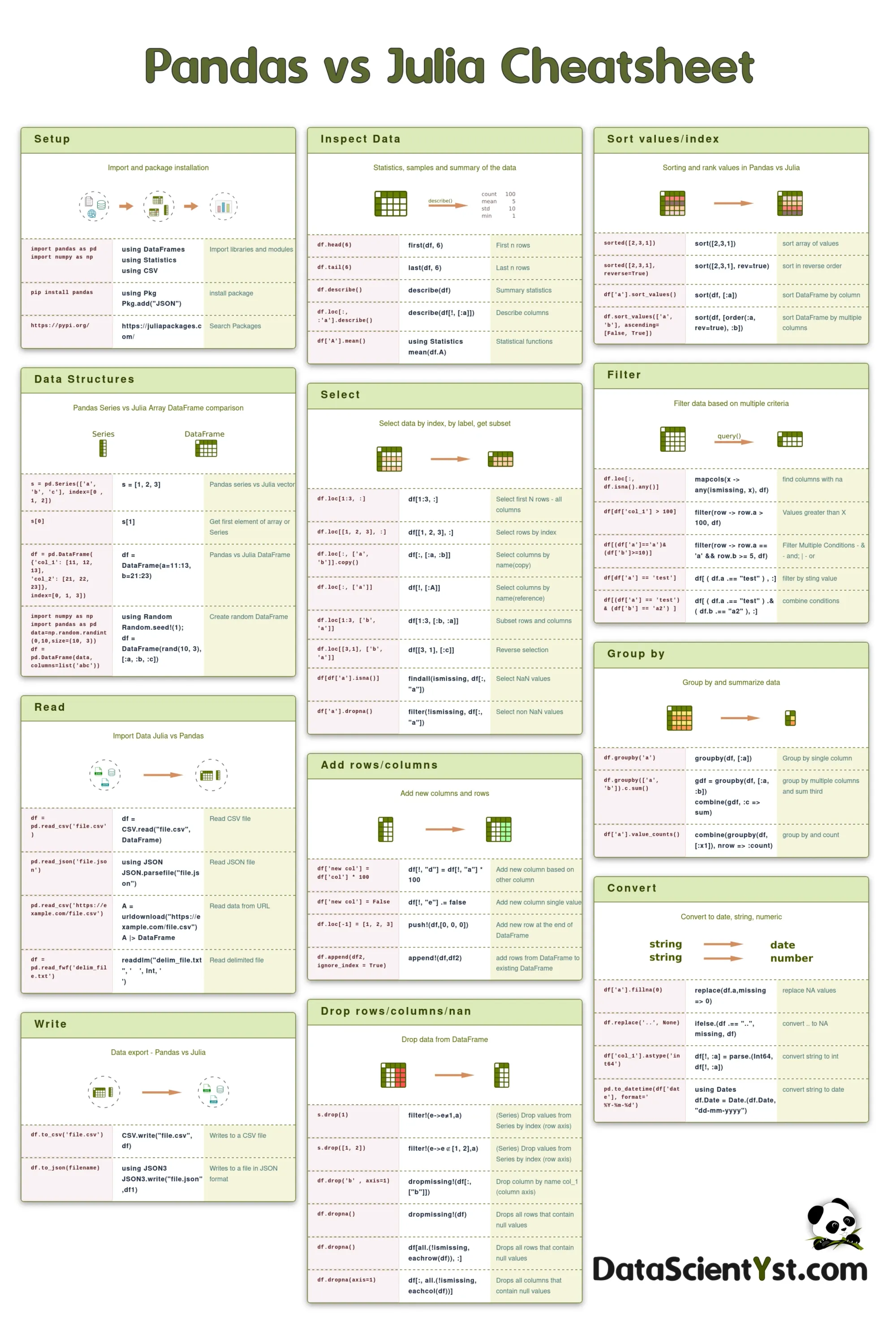This is a Python/Pandas vs Julia cheatsheet and comparison. You can find what is the equivalent of Pandas in Julia or vice versa. You can find links to the documentation and other useful Pandas/Julia resources.

The table below show the useful links for both:

Pandas Julia
data analysis tool high performance language
site https://pandas.pydata.org/ https://julialang.org/
docs https://pandas.pydata.org/docs/ https://docs.julialang.org/en/v1/
packages https://pypi.org/ https://juliapackages.com/
repo https://github.com/pandas-dev/pandas https://github.com/JuliaLang/julia

Below you can find equivalent code between Pandas and Julia. Have in mind that some examples might differ due to different indexing.

## Setup

Import and package installation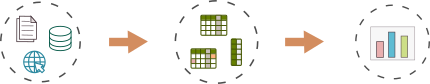```import pandas as pd import numpy as np```
using DataFrames using Statistics using CSV
Import libraries and modules
`pip install pandas`
install package
`https://pypi.org/`
https://juliapackages.com/
Search Packages

## Data Structures

Pandas Series vs Julia Array DataFrame comparison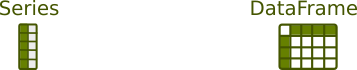`s = pd.Series(['a', 'b', 'c'], index=[0 , 1, 2])`
s = [1, 2, 3]
Pandas series vs Julia vector
`s`
s
Get first element of array or Series
```df = pd.DataFrame( {'col_1': [11, 12, 13], 'col_2': [21, 22, 23]}, index=[0, 1, 3])```
df = DataFrame(a=11:13, b=21:23)
Pandas vs Julia DataFrame
```import numpy as np import pandas as pd data=np.random.randint(0,10,size=(10, 3)) df = pd.DataFrame(data, columns=list('abc'))```
using Random Random.seed!(1); df = DataFrame(rand(10, 3), [:a, :b, :c])
Create random DataFrame

Import Data Julia vs Pandas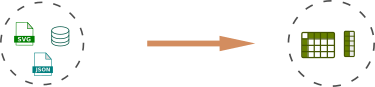`df = pd.read_csv('file.csv')`
`pd.read_json('file.json')`
using JSON JSON.parsefile("file.json")
`pd.read_csv('https://example.com/file.csv')`
`df = pd.read_fwf('delim_file.txt')`
readdlm("delim_file.txt", ' ', Int, ' ')

## Write

Data export - Pandas vs Julia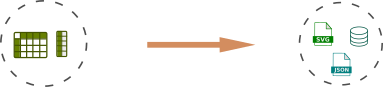`df.to_csv('file.csv')`
CSV.write("file.csv", df)
Writes to a CSV file
`df.to_json(filename)`
using JSON3 JSON3.write("file.json",df1)
Writes to a file in JSON format

## Inspect Data

Statistics, samples and summary of the data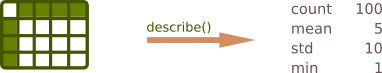`df.head(6)`
first(df, 6)
First n rows
`df.tail(6)`
last(df, 6)
Last n rows
`df.describe()`
describe(df)
Summary statistics
`df.loc[:, :'a'].describe()`
describe(df[!, [:a]])
Describe columns
`df['A'].mean()`
using Statistics mean(df.A)
Statistical functions

## Select

Select data by index, by label, get subset`df.loc[1:3, :]`
df[1:3, :]
Select first N rows - all columns
`df.loc[[1, 2, 3], :]`
df[[1, 2, 3], :]
Select rows by index
`df.loc[:, ['a', 'b']].copy()`
df[:, [:a, :b]]
Select columns by name(copy)
`df.loc[:, ['a']]`
df[!, [:A]]
Select columns by name(reference)
`df.loc[1:3, ['b', 'a']]`
df[1:3, [:b, :a]]
Subset rows and columns
`df.loc[[3,1], ['b', 'a']]`
df[[3, 1], [:c]]
Reverse selection
`df[df['a'].isna()]`
findall(ismissing, df[:, "a"])
Select NaN values
`df['a'].dropna()`
filter(!ismissing, df[:, "a"])
Select non NaN values`df['new col'] = df['col'] * 100`
df[!, "d"] = df[!, "a"] * 100
Add new column based on other column
`df['new col'] = False`
df[!, "e"] .= false
`df.loc[-1] = [1, 2, 3]`
push!(df,[0, 0, 0])
Add new row at the end of DataFrame
`df.append(df2, ignore_index = True)`
append!(df,df2)
add rows from DataFrame to existing DataFrame

## Drop rows/columns/nan

Drop data from DataFrame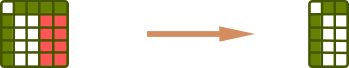`s.drop(1)`
filter!(e->e≠1,a)
(Series) Drop values from Series by index (row axis)
`s.drop([1, 2])`
filter!(e->e∉[1, 2],a)
(Series) Drop values from Series by index (row axis)
`df.drop('b' , axis=1) `
dropmissing!(df[:, ["b"]])
Drop column by name col_1 (column axis)
`df.dropna()`
dropmissing!(df)
Drops all rows that contain null values
`df.dropna()`
df[all.(!ismissing, eachrow(df)), :]
Drops all rows that contain null values
`df.dropna(axis=1)`
df[:, all.(!ismissing, eachcol(df))]
Drops all columns that contain null values

## Sort values/index

Sorting and rank values in Pandas vs Julia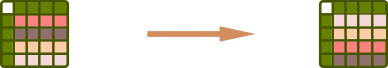`sorted([2,3,1])`
sort([2,3,1])
sort array of values
`sorted([2,3,1], reverse=True)`
sort([2,3,1], rev=true)
sort in reverse order
`df['a'].sort_values()`
sort(df, [:a])
sort DataFrame by column
`df.sort_values(['a', 'b'], ascending=[False, True])`
sort(df, [order(:a, rev=true), :b])
sort DataFrame by multiple columns

## Filter

Filter data based on multiple criteria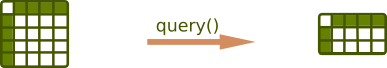`df.loc[:, df.isna().any()]`
mapcols(x -> any(ismissing, x), df)
find columns with na
`df[df['col_1'] > 100]`
filter(row -> row.a > 100, df)
Values greater than X
`df[(df['a']=='a')&(df['b']>=10)]`
filter(row -> row.a == 'a' && row.b >= 5, df)
Filter Multiple Conditions - & - and; | - or
`df[df['a'] == 'test']`
df[ ( df.a .== "test" ) , :]
filter by sting value
`df[(df['a'] == 'test') & (df['b'] == 'a2') ]`
df[ ( df.a .== "test" ) .& ( df.b .== "a2" ), :]
combine conditions

## Group by

Group by and summarize data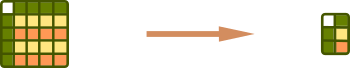`df.groupby('a')`
groupby(df, [:a])
Group by single column
`df.groupby(['a', 'b']).c.sum()`
gdf = groupby(df, [:a, :b]) combine(gdf, :c => sum)
group by multiple columns and sum third
`df['a'].value_counts()`
combine(groupby(df, [:x1]), nrow => :count)
group by and count

## Convert

Convert to date, string, numeric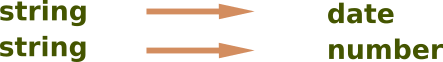`df['a'].fillna(0)`
replace(df.a,missing => 0)
replace NA values
`df.replace('..', None)`
ifelse.(df .== "..", missing, df)
convert .. to NA
`df['col_1'].astype('int64')`
df[!, :a] = parse.(Int64, df[!, :a])
convert string to int
`pd.to_datetime(df['date'], format='%Y-%m-%d')`
using Dates df.Date = Date.(df.Date, "dd-mm-yyyy")
convert string to date

## Install Julia Packages

To install new packages in Julia we can also use the Julia Package manager by:

• open Linux Terminal
• start Julia - `julia`
• Type `]` (right bracket). You don’t have to hit Return.
• Termimal will change to `(@v1.8) pkg>`
• you can provide the names of several packages separated by spaces.
• `Control-C` to exit the package manager

Example: `(v1.8) pkg> add JSON StaticArrays`

## Differences: Julia and Pandas

Pandas and Julia are both popular tools for data analysis and manipulation. Some key differences between them:

### Indexing

One big difference between Julia and Pandas is indexing:

### Syntax

Personally I prefer SQL syntax over both Julia and Pandas. I can work fine with both of them. As I have more experience with Python I would go with Pandas. Some people consider Julia to have better syntax since it was designed for data science. Example of syntax difference between Julia and Pandas:

``````# pandas
import pandas as pd
totals = df.groupby('product')['sales'].sum()

# julia
using DataFrames
using CSV
totals = combine(groupby(df, :product), :sales => sum)
``````

### Performance

In general Julia is faster for most operations and bigger datasets. For smaller datasets Pandas might be close or even better than Julia. The reason is for compilation time for Julia.

To test performance we can use dataset with 10M rows - Game Recommendations on Steam:

``````# pandas
%%time
import pandas as pd
df['hours'].mean()

# julia
@time begin
using CSV, DataFrames
df = CSV.File("recommendations.csv") |> DataFrame
result = mean(df[:, "hours"])
end
``````

The results are:

• Pandas
• CPU times: user 5.67 s, sys: 1.85 s, total: 7.52 s
• Wall time: 7.71 s
• Julia
• 7.257497 seconds (1.13 k allocations: 1.349 GiB, 2.63% gc time)

While for dataset - 12M rows we get:

• Pandas
• CPU times: user 34.8 s, sys: 3.74 s, total: 38.5 s
• Wall time: 42.4 s
• Julia
• 29.964544 seconds (162.12 M allocations: 9.878 GiB, 15.79% gc time)

First julia execution is slower so we take the second one.

### Libraries and Ecosystem

Pandas has a bigger community and ecosystem. The Python libraries offers greater variety of Packages in many areas:

• web scraping
• data science
• science
• etc

### Language Features

I prefer Julia for distributed computing and parallel computing. Pandas seems for me much better for visualization and EDA.

### Learning Curve

Again it depends on personal choice. Python is considered as one of the best programming languages for beginners. Julia surpassed Python in recent surveys for loved language:

stackoverflow survey - Most loved, dreaded, and wanted

Note: I need to add that I'm still learning and discovering Julia - so so statements above might change in future :)

## Pandas vs Julia docs

Pandas Julia
NA NA missing
Boolean False/True false/true
docs https://pandas.pydata.org/docs/ https://docs.julialang.org/en/v1/
packages https://pypi.org/ https://juliapackages.com/
repo https://github.com/pandas-dev/pandas https://github.com/JuliaLang/julia
basics https://pandas.pydata.org/docs/user_guide/basics.html https://docs.julialang.org/en/v1/base/punctuation/

## Summary

In summary, Pandas and Julia are both powerful tools for data analysis, but they have different strengths and weaknesses.

Pandas has a larger ecosystem of tools and is generally easier to learn. Julia is faster and has some unique language features that can make it more powerful for certain types of data analysis tasks.

Ultimately, the choice between Pandas and Julia depends on your specific requirements and preferences.

## Cheatsheet Image